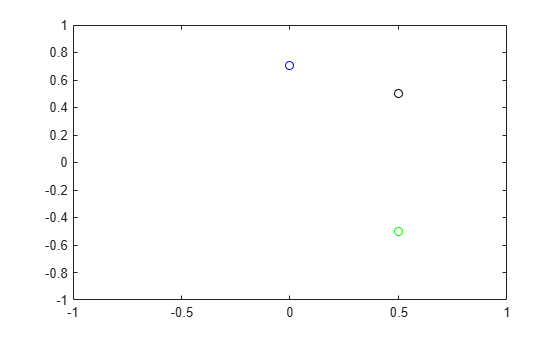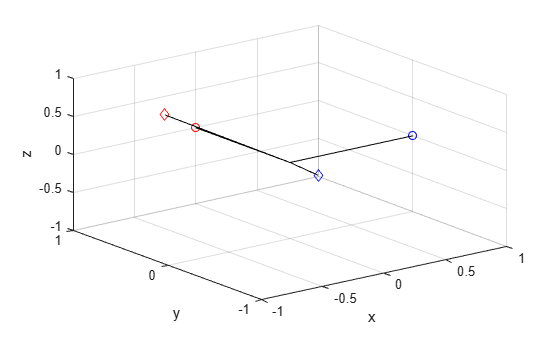rotatepoint

Quaternion point rotation

Description

example

rotationResult = rotatepoint(quat,cartesianPoints) rotates the Cartesian points using the quaternion, quat. The elements of the quaternion are normalized before use in the rotation.Examples

collapse all

Define a point in three dimensions. The coordinates of a point are always specified in order x, y, z. For convenient visualization, define the point on the x-y plane.

x = 0.5;
y = 0.5;
z = 0;

plot(x,y,'ko')
hold on
axis([-1 1 -1 1])Create a quaternion vector specifying two separate rotations, one to rotate the point 45 and another to rotate the point -90 degrees about the z-axis. Use rotatepoint to perform the rotation.

quat = quaternion([0,0,pi/4; ...
0,0,-pi/2],'euler','XYZ','point');

rotatedPoint = rotatepoint(quat,[x,y,z])
rotatedPoint = 2×3

-0.0000    0.7071         0
0.5000   -0.5000         0

Plot the rotated points.

plot(rotatedPoint(1,1),rotatedPoint(1,2),'bo')
plot(rotatedPoint(2,1),rotatedPoint(2,2),'go')Define two points in three-dimensional space. Define a quaternion to rotate the point by first rotating about the z-axis 30 degrees and then about the new y-axis 45 degrees.

a = [1,0,0];
b = [0,1,0];
quat = quaternion([30,45,0],'eulerd','ZYX','point');

Use rotatepoint to rotate both points using the quaternion rotation operator. Display the result.

rP = rotatepoint(quat,[a;b])
rP = 2×3

0.6124    0.5000   -0.6124
-0.3536    0.8660    0.3536

Visualize the original orientation and the rotated orientation of the points. Draw lines from the origin to each of the points for visualization purposes.

plot3(a(1),a(2),a(3),'bo');

hold on
grid on
axis([-1 1 -1 1 -1 1])
xlabel('x')
ylabel('y')
zlabel('z')

plot3(b(1),b(2),b(3),'ro');
plot3(rP(1,1),rP(1,2),rP(1,3),'bd')
plot3(rP(2,1),rP(2,2),rP(2,3),'rd')

plot3([0;rP(1,1)],[0;rP(1,2)],[0;rP(1,3)],'k')
plot3([0;rP(2,1)],[0;rP(2,2)],[0;rP(2,3)],'k')
plot3([0;a(1)],[0;a(2)],[0;a(3)],'k')
plot3([0;b(1)],[0;b(2)],[0;b(3)],'k')Input Arguments

collapse all

Quaternion that defines rotation, specified as a scalar quaternion, row vector of quaternions, or column vector of quaternions.

Data Types: quaternion

Three-dimensional Cartesian points, specified as a 1-by-3 vector or N-by-3 matrix.

Data Types: single | double

Output Arguments

collapse all

Rotated Cartesian points defined using the quaternion rotation, returned as a vector or matrix the same size as cartesianPoints.

Data Types: single | double

Algorithms

Quaternion point rotation rotates a point specified in R3 according to a specified quaternion:

${L}_{q}\left(u\right)=qu{q}^{*}$

where q is the quaternion, * represents conjugation, and u is the point to rotate, specified as a quaternion.

For convenience, the rotatepoint function takes in a point in R3 and returns a point in R3. Given a function call with some arbitrary quaternion, q = a + bi + cj + dk, and arbitrary coordinate, [x,y,z], for example,

rereferencedPoint = rotatepoint(q,[x,y,z])
the rotatepoint function performs the following operations:

1. Converts point [x,y,z] to a quaternion:

${u}_{q}=0+xi+yj+zk$

2. Normalizes the quaternion, q:

${q}_{n}=\frac{q}{\sqrt{{a}^{2}+{b}^{2}+{c}^{2}+{d}^{2}}}$

3. Applies the rotation:

${v}_{q}=q{u}_{q}{q}^{*}$

4. Converts the quaternion output, vq, back to R3

Extended Capabilities

Objects

Introduced in R2021a